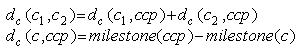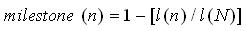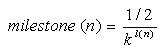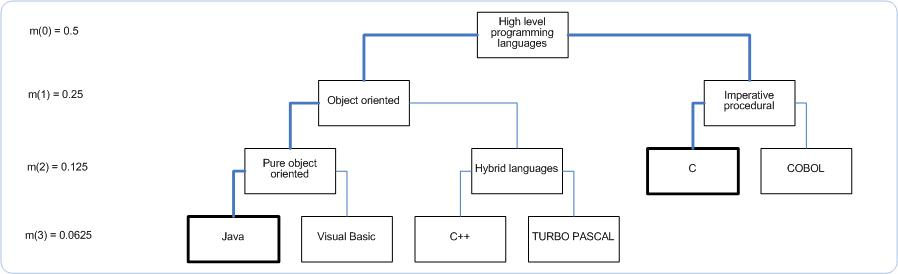# Implemented Matchers

November 2006

Matchers are Java classes which calculate the similarity of two object property values. Every matcher takes as input an RDFNode (property value) from a query graph and a semantically coresponding RDFNode from a resource graph. The output of a matcher is a similarity score of the two given nodes. SemMF provides three build-ing implementations of different matching techniques: taxonomic matcher, numeric matcher, and string matcher. In addition, users can create their own matchers and integrate them into SemMF.

##### Taxonomic Matcher

The taxonomic matcher is used if object property values are resources from a common taxonomy. The matcher computes the similarity between two concepts c 1 and c 2 based on the distance d(c1,c2) between them, which reflects their respective position in the concept hierarchy. The matcher is able to handle multiple inheritance of concepts at the leaf level of a taxonomy.

The concept similarity is defined as: sim(c1,c2) = 1 - d(c1,c2). Every concept in a taxonomy is assigned a milestone value. Since the distance between two given concepts in a hierarchy represents the path over the closest common parent (ccp), the distance is calculated as:The milestone values of concepts in a taxonomy are calculated with (as set in the matching description) either:

• a linear milestone calculator using the formula:
•where l(n) is the depth of the node n in the hierarchy and l(N) represents the deepest hierarchy level.

• or an exponential milestone calculator using the formula:
•where k is a factor greater than 1 indicating the rate at which the milestone values decrease along the hierarchy. It can be assigned different values depending on the hierarchy depth.

This formula implies two assumptions: the semantic difference between upper level concepts is greater than between lower level concepts (in other words: two general concepts are less similar than two specialized ones) and that the distance between brothers' is greater than the distance between parent' and child'.

USE CASE EXAMPLE:

As an example, we determine the distance between two concepts from a simple skill ontology: ' Java ' and 'C'. The picture below shows a snippet of the our simple taxonomy together with milestone values (with k=2) for the corresponding hierarchy levels (in brakets).Since the closest common parent is ' High level programming languages', the distance between ' Java ' and ' C ' is calculated as follows:

d (Java, C) = d (Java, High level programming languages) + d (C, High level programming languages)
= (0.5-0.0.0625) + (0.5-0.125)
= 0.8125

Consequently, the similarity between these two concepts equals: sim(Java, C) = 1  0.8125 = 0.1875

This value is logically much smaller than in the case of two more related concepts like Java and VisualBasic where their sim (Java, VisualBasic) = 0.875

The taxonomic matcher has one more parameter simInheritance which MAY be specified in a matching description. If turned to 'true' (default setting) then sim(queryConcept, resourceConcept = any descendant of queryConcept) = 1. This assumption seems to be reasonable because a subclass is always a kind of its superclass. Hovewer, there may be cases in which the actual distance between a subclass and its superclass should influence similarity calculation

##### NumericMatcher

This matcher is used to determine similarity of two numeric values. A good application example for this matching technique is the comparison of a product price some person is willing to pay (pq) with the actual product price (pr). For all pr > pq the similarity shall decrease with increasing pr. However, beyond a cerain value (upper bound) where pr would be inacceptably high the similarity shall equal 0. The numeric matcher has two parameters which MUST be specified in a matching description:

• decreaseSim - indicates whether sim(x,y) should decrease for y greater than x, smaller than x, or deviating from x in both directions. The values for this parameter are "upwards", "downwards", and "with deviation" respectively
• maxDevFraction - specifies the distance from x to an upper bound beyond which sim(x,y) equals 0. This distance is expressed as a fraction of x.

USE CASE EXAMPLE:

My optimum price for some product is 40\$. However, I might be ready to pay up to 50\$ for it. So, in order to customize the matcher for this example I set its parameters: decreaseSim="upwards" and maxDevFraction = 0.25 (becasue 0.25*40=10 is the distance to my upper bound = 50). A matcher configured this way would return: sim(40, 39) = 1, sim(40,44) = 0.6, sim(40,49) = 0.1, and sim(40, 55) = 0.

##### String Matcher

String matcher calculates similarity of two given RDFNodes based on their string serialization. Thus, in the case of Literals not only their lexical value but also their language and datatype are compared. Note that, this matcher only returns either 1 if both strings are equal or 0 i they are not. It has one parameter caseSensitive (= 'true' or 'false') which by default is set to 'false', thus indicating case INsensitive matching.